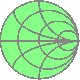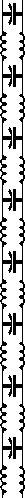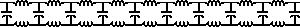n1ir covertFoolish Flyer# Fractal Antennas## Silvanus P. Thompson, F.R.S. this.fool's website of foolish fractals :

These pages are devoted to the analysis of those forms known as Fractals. The list of designs to the left cover topics reviewed by myself in the rec.radio.amateur.antenna newsgroup. The designs are those presented to the newsgroup through web site http://www.seanet.com/~rwclark/kb7qhc in the Feb.-mar. period of 1997. This work was moved to this present location in 1998. The next significant overhaul came in the summer of 1999 when all designs were re-evaluated in the Constant Wire and Constant Height surveys.

Introduction

Constant Wire survey

Constant Fractal survey

Constant Height survey

Constant Band survey

Wide Band survey

Constant Height Paired Fractals

Introduction

The goal of this work is to observe how fractal antennas might exhibit characteristics that are correlated to their form. To anticipate a question: "does a koch quadratic have more gain than a triadic?" The correlation inferred in this question is between the fractal iteration or form and the field distortions. This set-up question simplifies too much as there are a multitude of variables and constants that make simple answers to simple questions rare. In light of these impediments, the methods employed in these studies are designed to reduce variables and share constants. Through these methods, a number of tests in survey form were performed. Their purpose successfully achieved the goal where the data displays separable characteristics. This is demonstrated in the presentation of the "special wire equation" for any 2 iteration regular fractal.

There is no attempt to pre-qualify any of the designs except by the most fundamental relationship of fractal form, wavelength and length of wire. The height of an antenna or amount of wire consumed in the construction of any particular design was chosen to be an easily scalable and resolvable value. The designs here in these pages generally have a value of 16, 32, or 64 meters. Now this value may be an expression of the height of a design, or the amount of wire consumed (the length of wire in the bends and turns). Through these constraints in size, come reduction in variables.

The designs in this site are vertical for the greater majority with minor attention given to dipoles and loops. Another note, there is no attempt here to model against "real" conditions. All models are tested in as near-to-perfect circumstances as may be accomplished on a computer. There were several reasons for this choice and economy was high amongst them. By employing a perfect environment, the computations went much faster. Another consideration was that all work here was committed to the single goal of finding separable characteristics of fractals. The employment of a perfect environment presents no problems with that goal and also serves the requirements to reduce variables and share constants.

Other antennas offered singly as buttons to the left are amusing, interesting, useful, but not particularly insightful.

Constant Wire survey

The "constant wire" design is a survey of drive point impedance variation across a family of fractals, over a frequency range. The purpose of this testing is to reveal how fractal forms are differentiable through the resonant frequency for a constant length of wire. Increasing complexity in the underlying form shortens the antenna's height. In this page I derive what I call the fractal form's "ordering factor." By displaying all data (through all these pages) in the order derived on this page, the data's shape reveals each fractal form occupies a region distinct from the data of another fractal form. The data reveals the shift of the resonance is positively correlated to the number of elements in the fractal form. This is also illustrated in the Constant Band survey.

Two lengths are used in these surveys: 16 meters and 64 meters of wire consumed. Derived from these investigations are the "special wire equations" or mathematical formulas for determining the amount of wire consumed by any second iteration regular fractal. This portion of the page is still under construction.

Constant Fractal survey

This test looks at the variability of a fractal over iterations. Current under construction and it shows it by missing labels and links to some data.

Constant Height survey

The purpose of the "constant height" design is to look at fractal structures that use more wire than the monopole reference. The characteristic of interest here is with lobe gain.

There are two tables listing radiation characteristics for 16 Meter 2nd iteration designs. Compared to a simple monopole, the Fractals as a class offer more tunable opportunities. The proof of the is found with the Koch Quadratic providing a SWR null near or within every Amateur Band from 160M to 6M.

Constant Band survey

The intent behind this survey was to test for the possibility of distinguishing features at the first resonance. The key distinction found was that for a constant volume of wire in any fractal form, the first resonance changed, generally higher than a monopole cut with the same amount of wire. When the data is sorted by frequency, there is a clear trend where degree of complexity correlates to degree of resonance shift from the monopole standard.

Wide Band survey

This data reveals how fractals can become nearly flat matches over considerable stretches of frequency.

Constant Height Paired Fractals

This design is a whim where I combine two Koch Triadic forms in parallel. The twist though is that one branch is 1st iteration, and the other is 2nd iteration. I use an uncharacteristic 64 meters of wire in this experiment so the frequencies range in the 4 to 5 MHz range. Must be seen to be appreciated.

Insofar as whims go, this had an unanticipated consequence of demonstrating a folded monopole characteristic in the drive point Z.